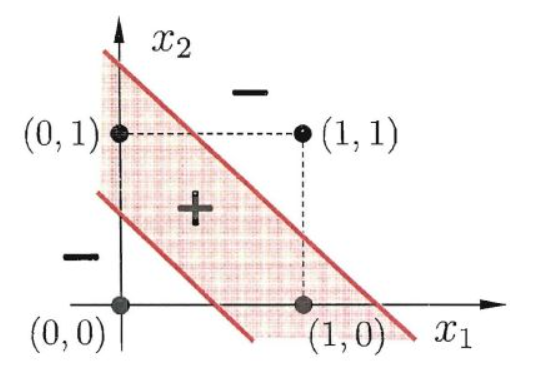# 一、感知机模型

$$f(x)=sign(w\cdot x+b)$$

# 二、感知机学习策略

## 2.3 学习算法

（1）选取初值$w_0,b_0$
（2）在训练集中选取数据$(x_i,y_i)$
（3）如果$y_i(w\cdot x_i+b)\le0$，更新参数$w=w+\eta y_i x_i$，$b=b+\eta y_i$，其中$\eta$为学习率，后项为两个参数的梯度
（4）重复上述过程，直到训练集中没有误分类点

# 三、习题

## 3.1 为什么感知机不能表示异或